QisBool Polygon & Path Functions

 QisBool_ConvertToCounterClockwise Objective To re-order the sequence of vertices in a polygon to be in counter clock-wise direction. Prototype void QisBool_ConvertToCounterClockwise(int* iXY, int iNV); Input Arguments a.     int* iXY: List of x,y co-ordinates for the polygon. (iNV*2 integers) b.     int iNV: Number of vertices in the polygon. (A rectangle has 5 vertices). Output Arguments a.     int* iXY: The newly oriented polygon is written to the same location as the original polygon. Return Value - Pre-Conditions - Operation a.     This function re-orders the vertices (iXY) so that they are in counter-clockwise direction. Post-Conditions - C++ Equivalent static void IQisBool::ConvertToCounterClockwise(int* iXY, int iNV); See Also -

 Objective To get the area of a polygon. Prototype double QisBool_GetPolygonArea(int* iXY, int iNV, void* iBooleanHandle); Input Arguments a.     int* iXY: List of x,y co-ordinates for the polygon. (iNV*2 integers) b.     int iNV: Number of vertices in the polygon. (A rectangle has 5 vertices). c.     void* iBooleanHandle: Handle to an instance of QisBool obtained using QisBool_Create. Output Arguments - Return Value a.     double: Area of the polygon in question. This area is unit less (number of grids) Pre-Conditions - Operation a.     This function computes the area of a polygon in terms of the number of grids. Since all QisBool co-ordinates are unit less (integer values), this area unit less too. Post-Conditions - C++ Equivalent virtual double IQisBool::GetPolygonArea(int* iXY, int iNV) = 0; See Also -

 Objective To convert a polygon into one or more convex polygons. Prototype int QisBool_PolyConvexing(   int* iXY, int iNV, int*** oXY, int** oNV, int* oN,   EConvexOutput iMode, int iValidateInput, void* iBooleanHandle ); Input Arguments a.     int* iXY: List of x,y co-ordinates for the polygon. (iNV*2 integers) b.     int iNV: Number of vertices in the polygon. (A rectangle has 5 vertices). c.     EConvexOutput iMode: eNO_CONVEX : There is no guarantee that the output polygons will be convex (default). eCONVEX_IN_X : The output polygons are guaranteed to be convex in X. eFULLY_CONVEX : The output polygons are guaranteed to be convex in X and Y. eTRAPEZOIDS : The output polygons are guaranteed to be trapezoids. d.     int iValidateInput: 1 (Perform validation of input) or 0 (Assume input is validated) e.     void* iBooleanHandle: Handle to an instance of QisBool obtained using QisBool_Create. Output Arguments a.     int*** oXY: Address of a double integer pointer which will point to a newly allocated list of x,y co-ordinates for the output convex polygons. b.     int** oNV: Address of an integer pointer which will point to a newly allocated list of vertex numbers for the output convex polygons. c.     int oN: Number of convex polygons generated. Return Value a.     success: 0 b.     failure: < 0. Call QisBool_ErrorMsg to get details. Pre-Conditions - Operation a.     This function converts a polygon into one or more set of convex polygons depending on the iMode setting. b.     If the polygon is already convex as per iMode, the output will have the same data as the input.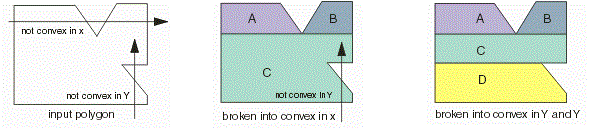Post-Conditions a.     The memory allocated by this function to store the output polygons must be release by calling QisBool_Release. C++ Equivalent virtual int IQisBool::PolyConvexing(   int* iXY, int iNV, int*** oXY, int** oNV, int* oN,   EConvexOutput iMode, bool iValidateInput ) = 0; See Also

 Objective To convert round paths to boundaries. Prototype int QisBool_RoundPathToBoundaries(   int* iXY, int iNV, int iWidth, int*** oXY, int** oNV, int* oN,   short iArcRes, double iArcSag, void* iBooleanHandle ); Input Arguments a.     int* iXY: A list of x,y co-ordinates of the path to be converted. b.     int iNV: Number of vertices in the path. c.     int iWidth: Width of the path. d.     short iArcRes: The acceptable arc resolution of round corner of the output boundary. e.     double iArcSag: The acceptable chord error of the round corner of the output boundary. f.      void* iBooleanHandle: Handle to an instance of QisBool obtained using QisBool_Create.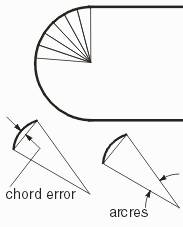Output Arguments a.     int*** oXY: Address of a double integer pointer which will point to a newly allocated list of x,y co-ordinates for the output boundaries. b.     int** oNV: Address of an integer pointer which will point to a newly allocated list of vertex numbers for the output boundaries. c.     int oN: Number of output boundaries generated. Return Value a.     success: 0 b.     failure: < 0. Call QisBool_ErrorMsg to get details. Pre-Conditions a.     If both iArcRes and iArcSag are both specified, the parameter with a finer resolution is user. Operation a.     This function converts a round path to a set of boundaries, one boundary per segment of the path.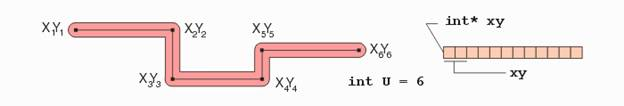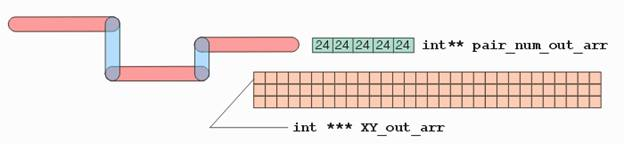Post-Conditions a.     The memory allocated by this function to store the output polygons must be release by calling QisBool_Release. C++ Equivalent virtual int IQisBool::RoundPathToBoundaries(   int* iXY, int iNV, int iWidth, int*** oXY, int** oNV, int* oN,   short iArcRes, double iArcSag ) = 0; See Also

 Objective To group connected boundaries into sets. Prototype int QisBool_GroupConnectedPolygons(   int** iXY, int* iNV, int iN, int*** oXY, int** oNV, int* oN,   void* iBooleanHandle ); Input Arguments a.     int** iXY: A list of x,y co-ordinates of the boundaries to be grouped. b.     int* iNV: A list of number of vertices for each of those boundaries. c.     int iN: Number of input boundaries. d.     void* iBooleanHandle: Handle to an instance of QisBool obtained using QisBool_Create. Output Arguments a.     int*** oXY: Address of an integer double pointer that will point to a newly allocated list of index numbers (0 to iN-1) per set of connected polygons. b.     int** oNV: Address of an integer pointer that will point to a newly allocated list of number of indices per set of connected polygons. c.     int oN: Number of sets of connected polygons. Return Value a.     success: 0 b.     failure: < 0. QisBool_ErrorMsg to get details. Pre-Conditions - Operation a.     This function analyses all of the input polygons (iN, iNV, iXY) and groups them into oN sets based on connectivity. Any two polygons from the input set that are geometrically connected fall into the same set. b.     Each set is represented by a list of indices corresponding to the polygons belonging to that set. An index to a polygon in the input set is simply an integer from 0 to iN-1 that contains the data corresponding to that polygon in iNV and iXY. If I is an index to a polygon in the input set, iNV[I] stores the number of vertices belonging to that polygon and iXY[I] points to iNV[I]*2 integers representing it's x,y co-ordinates. Post-Conditions a.     The memory allocated to store the sets must be released using QisBool_Release. C++ Equivalent virtual int IQisBool::GroupConnectedPolygons(   int** iXY, int* iNV, int iN, int*** oXY, int** oNV, int* oN ) = 0; See Also

 Objective To convert a path (flush or half extended) to boundaries. Prototype int QisBool_Path2Boundaries(   EPathType iType, int iWidth, int* iXY, int iNV,   int*** oXY, int** oNV, int* oN,   int iUnionize, void* iBooleanHandle ); Input Arguments a.     int* iXY: A list of x,y co-ordinates of the path to be converted. b.     int iNV: Number of vertices in the path. c.     int iWidth: Width of the path. d.     int iUnionize: 1 (Unionize the various segments of the path as one boundary) or 0 (Generate a separate boundary for each segment of the path). e.     EPathType iType: Type of path eFLUSH_PATH : flush path eHALFEXT_PATH : half extended path f.      void* iBooleanHandle: Handle to an instance of QisBool obtained using QisBool_Create. Output Arguments a.     int*** oXY: Address of a double integer pointer which will point to a newly allocated list of x,y co-ordinates for the output boundaries. b.     int** oNV: Address of an integer pointer which will point to a newly allocated list of vertex numbers for the output boundaries. a.     int oN: Number of output boundaries generated. Return Value a.     success: 0 b.     failure: < 0. Call QisBool_ErrorMsg to get details. Pre-Conditions a.     Only flush and half extended paths can be converted using this function. b.     To convert round paths use QisBool_RoundPathToBoundaries. Operation a.     This function converts a flush/half extended path to boundaries. b.     If iUnionize is set, the path is converted to one boundary, otherwise a boundary is generated for each segment of the path.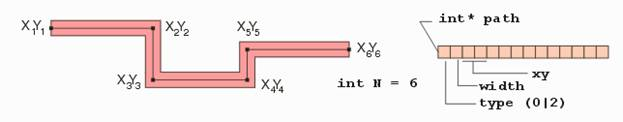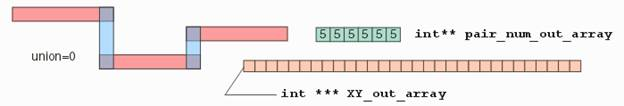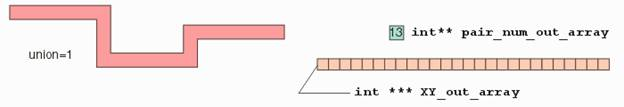Post-Conditions a.     The memory allocated by this function to store the output polygons must be release by calling QisBool_Release. C++ Equivalent virtual int IQisBool::Path2Boundaries(   EPathType iType, int iWidth, int* iXY, int iNV,   int*** oXY, int** oNV, int* oN, bool iUnionize ) = 0; See Also

 QisBool_BreakPolygons Objective To limit the number of vertices in the input polygons by breaking those that exceed a specified vertex count. Prototype int QisBool_BreakPolygons(   int** iXY, int* iNV, int iN, int iMaxPts,   int*** oXY, int** oNV, int* oN, int iValidate, void* iBoolHandle ); Input Arguments a.     int** iXY: A list of x,y co-ordinates of the input polygons. (iN integer arrays) b.     int* iNV: A list of number of vertices in the input polygons. (iN integers) c.     int iN: Number of input polygons. d.     int iMaxPts: Maximum number of vertices expected. e.     int iValidate: 1 (Validate input polygons) 0 (Assume the polygons have been validated) f.      void* iBooleanHandle: Handle to an instance of QisBool obtained using QisBool_Create. Output Arguments a.     int* oN: Address of an integer to store the number of polygons in the output. b.     int** oNV: Address of an integer pointer that will point to a newly allocated list of number of vertices for each of the output polygons. (contains oN integers). If I is the index of an output polygon (0 <= I < *oN), then (*oNV)[I] represents the number of vertices for that polygon. c.     int*** oXY: Address of an integer double pointer that will point to a new allocated list of x,y co-ordinates for each of the output polygons. (contains oN integer arrays). If I is the index of an output polygon (0 <= I < *oN), then (*oXY)[I] is an integer array of (*oNV)[I] * 2 integers representing the x,y co-ordinates of that polygon. Return Value a.     success: 0 b.     failure: < 0. Call QisBool_ErrorMsg to get details. Pre-Conditions a.     If iValidate is 0, it is assumed that the input polygons are legal and non-re-entrant. Operation a.     This functions breaks any input polygon which has more vertices than iMaxPts.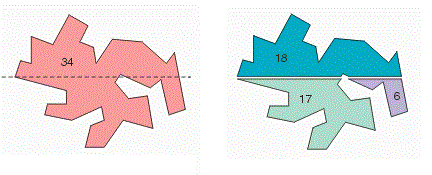Post-Conditions a.     The memory allocated by QisBool to store the output polygons (oN, oNV, oXY) must be released using QisBool_Release. C++ Equivalent virtual int IQisBool::BreakPolygons(   int** iXY, int* iNV, int iN, int iMaxPts,   int*** oXY, int** oNV, int* oN, bool iValidate ) = 0; See Also

 Objective To limit the number of vertices in the input polygons by breaking those that exceed a specified vertex count and get the edges used to break the polygons. Prototype int QisBool_BreakPolygonGetEdges(   int** iXY, int* iNV, int iN, int iMaxPts,   int*** oXY, int** oNV, int* oN, bool iValidate,   int** oEdgeArr, int* oNEdges, void* iBoolHandle ); Input Arguments a.     int** iXY: A list of x,y co-ordinates of the input polygons. (iN integer arrays) b.     int* iNV: A list of number of vertices in the input polygons. (iN integers) c.     int iN: Number of input polygons. d.     int iMaxPts: Maximum number of vertices expected. e.     int iValidate: 1 (Validate input polygons) 0 (Assume the polygons have been validated) f.      void* iBooleanHandle: Handle to an instance of QisBool obtained using QisBool_Create. Output Arguments a.     int* oN: Address of an integer to store the number of polygons in the output. b.     int** oNV: Address of an integer pointer that will point to a newly allocated list of number of vertices for each of the output polygons. (contains oN integers). If I is the index of an output polygon (0 <= I < *oN), then (*oNV)[I] represents the number of vertices for that polygon. c.     int*** oXY: Address of an integer double pointer that will point to a new allocated list of x,y co-ordinates for each of the output polygons. (contains oN integer arrays). If I is the index of an output polygon (0 <= I < *oN), then (*oXY)[I] is an integer array of (*oNV)[I] * 2 integers representing the x,y co-ordinates of that polygon. d.     int* oNEdges: Address of an integer to store the number of edges. e.     int** oEdgeArr: Address of an integer pointer that will point to a newly allocated list of edges. This list will contain (*oNEdges) * 4 integers. (*oEdgeArr)[0,1] represents the x,y co-ordinates of the start point of the first edge. (*oEdgeArr)[2,3] represents the x,y co-ordinates of the end point of the first edge and so on. Return Value a.     success: 0 b.     failure: < 0. Call QisBool_ErrorMsg to get details. Pre-Conditions a.     If iValidate is 0, it is assumed that the input polygons are legal and non-re-entrant. Operation a.     This functions breaks any input polygon which has more vertices than iMaxPts and returns a list of edges introduced to break the polygons.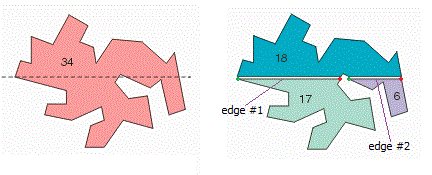Post-Conditions a.     The memory allocated by QisBool to store the output polygons (oNV, oXY) must be released using QisBool_Release. b.     The memory allocated by QisBool to store the edges (oEdgeArr) must be released using QisBool_ReleaseArray. C++ Equivalent int IQisBool::BreakPolygonGetEdges(   int** iXY, int* iNV, int iN, int iMaxPts,   int*** oXY, int** oNV, int* oN, bool iValidate,   int** oEdgeArr, int* oNEdges ) = 0; See Also QisBool_Release

 Objective To size a polygon using standard sizing. Prototype int QisBool_PolyCompensation(   int* iXY, int iNV, double iSizing, int** oXY , int* oNV, void* iBoolHandle ); Input Arguments a.     int iNV: Number of vertices in the input polygon. b.     int* iXY: x,y co-ordinates of the input polygon (iNV * 2 integers) c.     double iSizing: Amount of sizing to be applied in both X and Y. d.     void* iBooleanHandle: Handle to an instance of QisBool obtained using QisBool_Create. Output Arguments a.     int* oNV: Address of an integer to store the number of vertices in the output polygons. b.     int** oXY: Address of an integer pointer that will point to a newly allocated array representing the x,y co-ordinates of the output polygon. ( (*oNV) * 2 integers ) Return Value a.     success: 0 b.     failure: < 0. Call QisBool_ErrorMsg to get details. Pre-Conditions a.     This function uses a very basic form of sizing. Therefore it must be used only with very simple non-reentrant manhattan data. b.     The output is not guaranteed to be a legal polygon if the input is not simple. Operation a.     This function sizes the input polygon by iSizing amount in X and Y using a very basic sizing technique.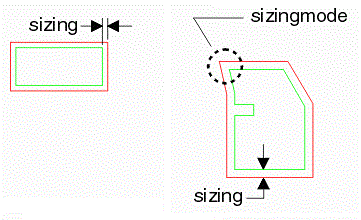Post-Conditions a.     The memory allocated to store the x,y co-ordinates of the output polygon (oXY) must be released using QisBool_ReleaseArray. C++ Equivalent virtual int IQisBool::PolyCompensation(   int* iXY, int iNV, double iSizing, int** oXY , int* oNV ) = 0; See Also

 417 Ingalls St. Santa Cruz CA 95060 [T] +1 831-426-6163 [F] +1 831-426-2824 [E] info@artwork.com